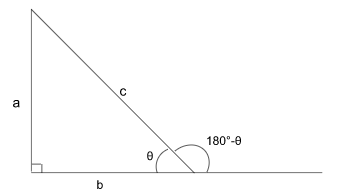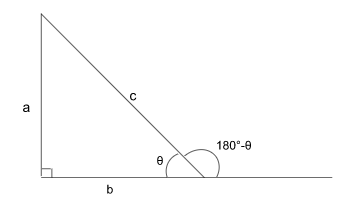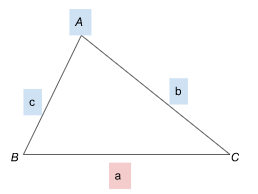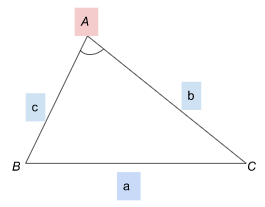Study S3 Mathematics Further Trigonometry - Geniebook# Further Trigonometry

• Sine and Cosine of obtuse angles
• Finding area of triangle using trigonometry
• Sine Rule
• Cosine Rule

## 1. Sine Of Obtuse Angles\begin{align*} sin\; θ = sin(180°-θ) \end{align*}

We know that,

\begin{align*} \textstyle ​​sin \;\theta &= \frac {opposite} {hypotenuse} ​​\\ \\ \therefore \;\;\;\;\;\;\;\;\;\;\; sin \; θ &= \frac {a} {c} \\ \\ ​​sin \;(180^\circ -\;θ) &= sin \;θ \\ \\ &=\frac {a} {c} \\ \end{align*}

## 2. Cosine Of Obtuse Angle$$cos \;θ = - cos(180°- θ)$$

We know that,

\begin{aligned} cos \;θ &= \frac {b}{c} \\ \\ cos(180°-θ) &= - cos \;θ \\ \\ &= - \frac {b}{c} \end{aligned}

## 3. Area Of Triangle

The above mentioned triangle is not a right angle triangle and hence its area cannot be given by the conventional formula.

$$\text{Area Of △ABC} = \frac {1}{2} ab \;sin\;C$$

## 4. Sine Rule

Sine rule gives us

\begin{aligned} \frac {sin \;A}{a} = \frac {sin \;B}{b} = \frac {sin \;C}{c}\\ \end{aligned}

or

\begin{aligned} \frac {a}{sin \;A} = \frac {b}{sin \;B} = \frac {c}{sin \;C}\\ \end{aligned}

The smaller letters signify the length of the side whereas the capital letters indicate the angles.

The side(s) correspond opposite to the angle(s) given, and the question will always have at least one of the sides or angles mentioned in it.

The similar coloured letters are always paired together.

## 5. Cosine Rule

Case 1:

In this situation we usually find the length of the side when 2 sides and 1 included angle is given.In this situation, we need to find the value of $$a$$ when values of $$b$$, $$c$$ & $$A$$ are given to us.

You can use the following formula to obtain the value of $$a$$

\begin{aligned} a^2 &= b^2 + c^2- 2bc \;cos\;A \\ \\ \implies \;\;\; a &= \sqrt {b^2 + c^2 - 2bc \; cosA} \end{aligned}

Case 2:

In this situation we are expected to find the angle when the values of $$3$$ sides are given to us.Taking the example here, we are given the values of $$a,b,c$$ and the value of angle $$A$$ is to be found. In this case we use the formula,

\begin{aligned} cosA &= \frac {b^2 + c^2 - a^2} {2bc} \\ \\ \implies \qquad A &= cos^{-1} ( \frac {b^2 + c^2 - a^2} {2bc} ) \end{aligned}

Example 1:

The figure shows Quadrilateral $$ABCD$$, where $$AB$$ is parallel to $$DC$$.

Find

1. the length of $$AC$$
2. angle $$ADC$$Solution:

For Part (A):

When we look at the $$△ADC$$ we do not have sufficient information, since we need to know at least three things to go ahead with our calculations.

However upon looking at $$△ABC$$ we see there are three parameters given to us, that is two sides and $$1$$ included angle which satisfies the condition for case 1 in Sine Rule and hence, we can use the formula   $$a^2= b^2+ c^2- 2bc \;cosA$$ to find out the length of $$AC$$.

Hence,

\begin{align*} AC &= \sqrt {6^2+ 5^2- 2 \times 6 \times 5 \times cos \;53°} \\ \\ AC &=4.989 \;cm ≈ 4.99\;cm \end{align*}

For Part (B):

Now that we have the length of 2 sides and an angle you can use the sine rule to determine the value of Angle $$ADC$$

\begin{align*} \frac {sin ∠ADC}{AC} &= \frac {sin ∠DAC}{CD}\\ \\ \implies \frac {sin ∠ADC} {4.989} &= \frac {sin 82°}{7} \\ \\ \implies \;sin ∠ADC &= \frac {4.989 \;sin \;82°} {7} \\ \\ \implies \;\;\;\;\;\;∠ADC &= sin^{-1} \bigg( \frac {4.989 \;sin \;82°} {7} \bigg) \\ \\ \implies \;\;\;\;\;\;∠ADC &= 44.893° ≈ 44.9° \\ \end{align*}

## Conclusion

In this article, we have discussed formulas relating to Sine & Cosine of Obtuse Angles, and the  concepts and techniques on how to find the area of a triangle using trigonometry, the formulae for Sine Rule and Cosine Rule and ways to apply them in various situations.

Continue Learning
Further Trigonometry Quadratic Equations And Functions
Linear Inequalities Laws of Indices
Coordinate Geometry Graphs Of Functions And Graphical Solution
Applications Of TrigonometryPrimaryPrimary 1Primary 2Primary 3Primary 4Primary 5Primary 6SecondarySecondary 1Secondary 2Secondary 3PhysicsChemistryMathsE-Maths
Further Trigonometry
Linear Inequalities
Laws Of Indices
Coordinate Geometry
Graphs Of Functions And Graphical Solution
Applications Of TrigonometryA-MathsSecondary 4